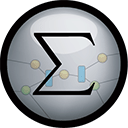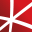# Top 20 NuGet math Packages

Math.NET Numerics is the numerical foundation of the Math.NET project, aiming to provide methods and algorithms for numerical computations in science, engineering and every day use. Supports .Net Framework 4.0 or higher and .Net Standard 1.3 or higher, on Windows, Linux and Mac.
Runtime expressions parser and evaluator (formulas, conditions, method calls, properties/indexers etc). Builds LINQ expression tree and compiles it to lambda delegate with full support of dynamically typed variables.## Math.NET Numerics - Strong Name Edition

Math.NET Numerics is the numerical foundation of the Math.NET project, aiming to provide methods and algorithms for numerical computations in science, engineering and every day use. Supports .Net Framework 4.0 or higher and .Net Standard 1.3 or higher, on Windows, Linux and Mac. This package contain...
.NET wrapper for the Operations Research Tools project
F# Modules for Math.NET Numerics, the numerical foundation of the Math.NET project, aiming to provide methods and algorithms for numerical computations in science, engineering and every day use. Supports .Net Framework 4.5 or higher and .Net Standard 1.6 or higher, on Windows, Linux and Mac.
Math.NET Symbolics is a basic open source computer algebra library for .Net and Mono. Written in F# but works well in C# as well. Supports .Net Framework 4.5 or higher and .Net Standard 2.0 or higher, on Windows, Linux and Mac.## Math.NET Numerics for F# - Strong Name Edition

F# Modules for Math.NET Numerics, the numerical foundation of the Math.NET project, aiming to provide methods and algorithms for numerical computations in science, engineering and every day use. Supports .Net Framework 4.5 or higher and .Net Standard 1.6 or higher, on Windows, Linux and Mac. This pa...
NumSharp is the fundamental library for scientific computing with .NET providing a similar API to python's numpy scientific library. NumSharp has full N-D, broadcasting and axis support. If you want to use .NET to get started with machine learning, NumSharp will be your best tool.
The OPTANO Modeling library allows you to use C# as a Modeling language for mathematical optimization (mixed integer programming (MIP) and linear programming (LP)). It has a lightweight footprint and connects to several solvers.
A Simple Math and Pseudo C# Expression Evaluator in One C# File. Can also execute small C# like scripts
The Xamarin.Forms front end for CSharpMath.
A library for advanced numerical computing, with support for data wrangling, statistics, matrix algebra, advanced functions, numerical integration and optimization, and Fourier transforms.## mXparser – Math Expressions Parser / Formula Evaluator library for JAVA Android C# .NET/MONO (.NET Framework, .NET Core, .NET Standard, .NET PCL, Xamarin.Android, Xamarin.iOS) CLS compliant

mXparser is a super easy, rich, fast and highly flexible math expression parser library (parser and evaluator of mathematical expressions / formulas provided as plain text / string). Software delivers easy to use API for JAVA, Android and C# .NET/MONO (Common Language Specification compliant: F#, Vi...
Foundational classes for financial, engineering, and scientific applications, including complex number classes, general vector and matrix classes, structured sparse matrix classes and factorizations, general sparse matrix classes and factorizations, general matrix decompositions, least squares solut...
The GPU-accelerated version of package CenterSpace.NMath. With a few minor exceptions, such as optional GPU configuration settings, the API is identical between CenterSpace.NMath.Premium and CenterSpace.NMath. If using at least .NET Framework 4.6.1 or .NET Core 2.0, we recommend using one of our NMa...## NMath - Standard Library - Windows x64

Foundational classes for financial, engineering, and scientific applications, including complex number classes, general vector and matrix classes, structured sparse matrix classes and factorizations, general sparse matrix classes and factorizations, general matrix decompositions, least squares solut...
Foundational classes for financial, engineering, and scientific applications, including complex number classes, general vector and matrix classes, structured sparse matrix classes and factorizations, general sparse matrix classes and factorizations, general matrix decompositions, least squares solut...
xFunc.Maths is a simple and easy to use library that allows you to build mathematical expressions.
The Extreme Optimization Numerical Libraries for .NET are a set of libraries for numerical computing and data analysis. This is the main package that contains all the core functionality. For optimal performance, we strongly recommend also referencing one of the native packages based on Intel's Mat...
Jace.NET is a high performance calculation engine for the .NET platform that can dynamically parse, interprete and execute strings containing mathematical functions. Jace.NET is available for the various .NET flavors: .NET, .NET Core, WinRT, Xamarin.Android and Xamarin.IOS.